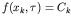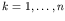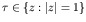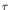Computational & Technology Resources an online resource for computational,engineering & technology publications not logged in - login Civil-Comp ProceedingsISSN 1759-3433 CCP: 84PROCEEDINGS OF THE FIFTH INTERNATIONAL CONFERENCE ON ENGINEERING COMPUTATIONAL TECHNOLOGY Edited by: B.H.V. Topping, G. Montero and R. Montenegro Paper 30Computing Rational Gauss-Chebyshev Quadrature Formulas with Complex Poles K. Deckers, J. Van Deun and A. BultheelDepartment of Computer Science, Catholic University of Leuven, Belgium doi:10.4203/ccp.84.30 Full Bibliographic Reference for this paper K. Deckers, J. Van Deun, A. Bultheel, "Computing Rational Gauss-Chebyshev Quadrature Formulas with Complex Poles", in B.H.V. Topping, G. Montero, R. Montenegro, (Editors), "Proceedings of the Fifth International Conference on Engineering Computational Technology", Civil-Comp Press, Stirlingshire, UK, Paper 30, 2006. doi:10.4203/ccp.84.30 Keywords: quadrature formulas, orthogonal rational functions, complex poles, algorithm, numerical integration, Gauss-Chebyshev. Summary This paper provides a fast algorithm to compute arbitrarily many nodes and weights for the rational Gauss-Chebyshev quadrature formulas integrating exactly in spaces of rational functions with arbitrary complex poles outside [-1,1] . The algorithm is based on the derivation of explicit expressions for the Chebyshev (para-)orthogonal rational functions . Once the nodes are known, the computation of the weights is straightforward. These nodes cannot be found analytically but have to be solved numerically (using Newton's method) from the equation,, whereis a parameter originating from the expression of the para-orthogonal rational function, mapping the last pole in the sequence (if not real) on the extended real line outside [-1,1], Ck is a constant only depending on the node xk to be computed and f is a smooth, monotonically increasing function that depends on the first n poles. Some characteristics of this function were already given in  for the case of all real poles, and two methods, namely linear extrapolation (LE) and asymptotic zero distribution (AZD), were derived for determining a sequence of initial values for the nodes, assuring convergence of Newton's method. We give a thorough analysis of this function in the case of complex poles, based on their distribution in the complex plane, to investigate whether these characteristics still hold. These characteristics cannot be extended to the entire complex plane, but at least to a part of it. Furthermore, restricted to the part of the complex plane where these characteristics do not hold, we examine the distribution of the interior local maxima of the first derivative of f when considering the worst distribution of the poles possible, i.e. when the poles in the sequence come closer to the boundary [-1,1]. Based on this analysis, we investigate whether (monotonic) convergence of Newton's method can be assured when starting from the initial values determined by using LE or AZD, and look at some general advantages and disadvantages of each method. The main advantage of AZD is that all the initial values can be determined at the same time, so that the Newton iterations can be done simultaneously for all the nodes, using matrix operations. Secondly, convergence of Newton's method can be expected, but not necessarily always assured, for sequences containing poles not too close to the boundary. But a disadvantage of AZD is that it does not work well for sequences containing poles close to the boundary. When using LE, the initial values have to be determined serially and when computing the previous node, convergence is essential so that the computation can be continued for the next node. Because of this, LE is more interesting in combination with AZD when some but not all nodes can be computed with the latter, rather than as a method on its own. Nevertheless, LE has an advantage over AZD for sequences of different poles, because under certain conditions on the distribution of the poles it converges monotonically to the exact solution. For sequences containing poles close to the boundary, Newton's method does not always converge when using AZD alone or combined with LE, because of a peak shaped interior local maxima of the first derivative of f. For this reason we derive a new method based on the distribution of these interior local maxima, called asymptotic inflection point distribution (AIPD). Through estimating the local maxima we try to find a sequence of initial values for the nodes, assuring monotonic convergence for sequences containing only poles close to the boundary, rather than trying to find initial values close to the exact solution. For sequences containing poles close to the boundary as well as poles away from the boundary, Newton's method does not always converge when using AIPD alone or combined with LE, so that other combinations (like for instance combining AZD with AIPD) need to be considered. The computations for these combinations are more difficult to organise than for the former combinations and are open to further research. Many observations strongly indicate that, when considering more combinations of methods, in all cases under consideration Newton's method converges to the exact solutions. In some exceptional cases, a few additional iterations using the method of bisection are required to obtain full precision. Another unresolved problem is determining the value for the parameter(in case the last pole in the sequence is not real) so that the computations of the nodes are optimal. References 1 Van Deun, J. and A. Bultheel, "Orthogonal rational functions and quadrature on an interval", Journal of Computational and Applied Mathematics, 153(1-2), 487-495, 2003. doi:10.1016/S0377-0427(02)00598-8 2 Deckers, K. and J. Van Deun and Bultheel, A., "Rational Gauss-Chebyshev quadrature formulas for complex poles outside [-1,1]", Report TW 448, Department of Computer Science, Catholic University of Leuven, 2006. 3 Van Deun, J. and A. Bultheel and González Vera, P., "On computing rational Gauss-Chebyshev quadrature formulas", Mathematics of Computation, 75, 307-326, 2006. doi:10.1090/S0025-5718-05-01774-6 purchase the full-text of this paper (price £20) Back to top ©Civil-Comp Limited 2020 - terms & conditions